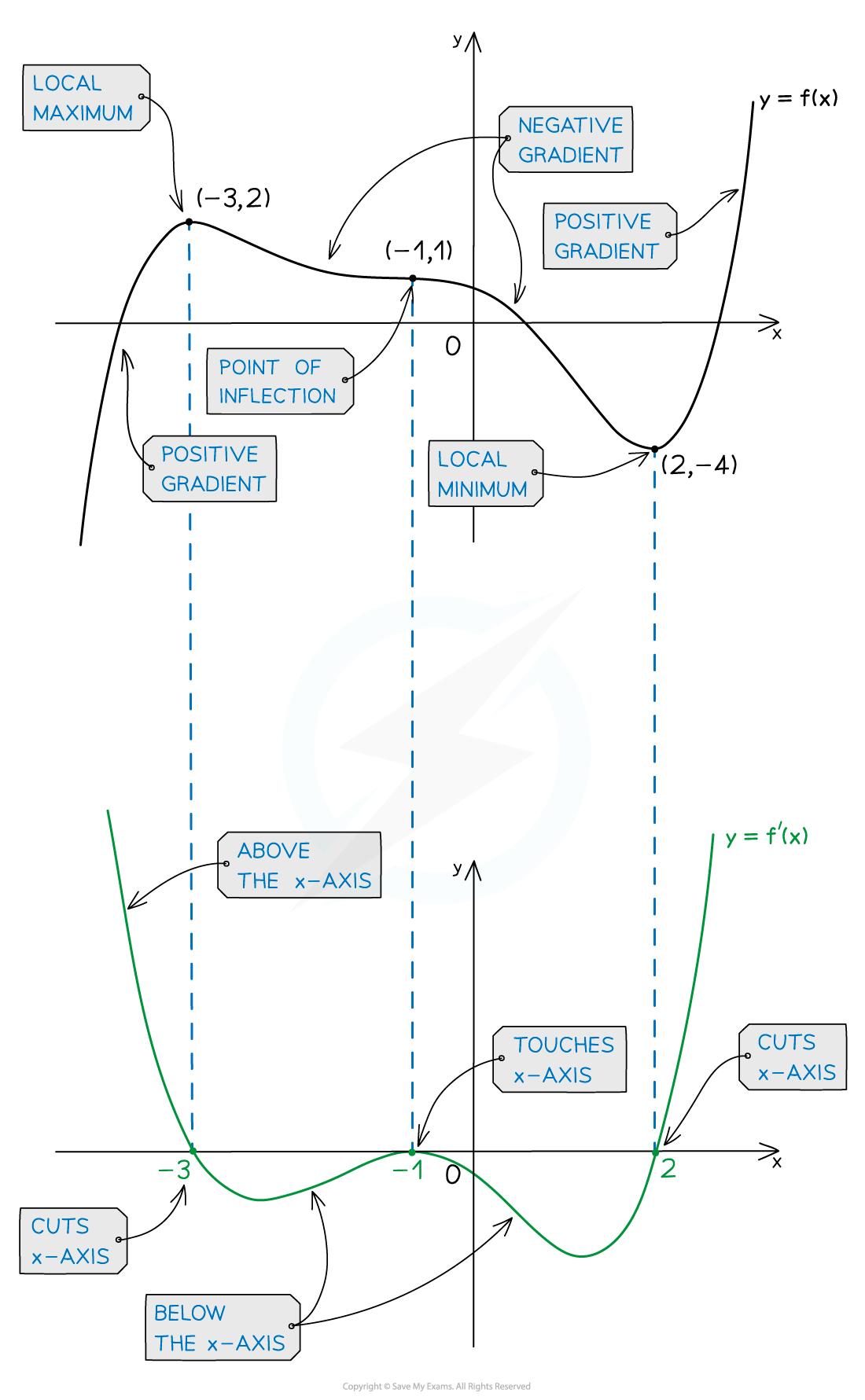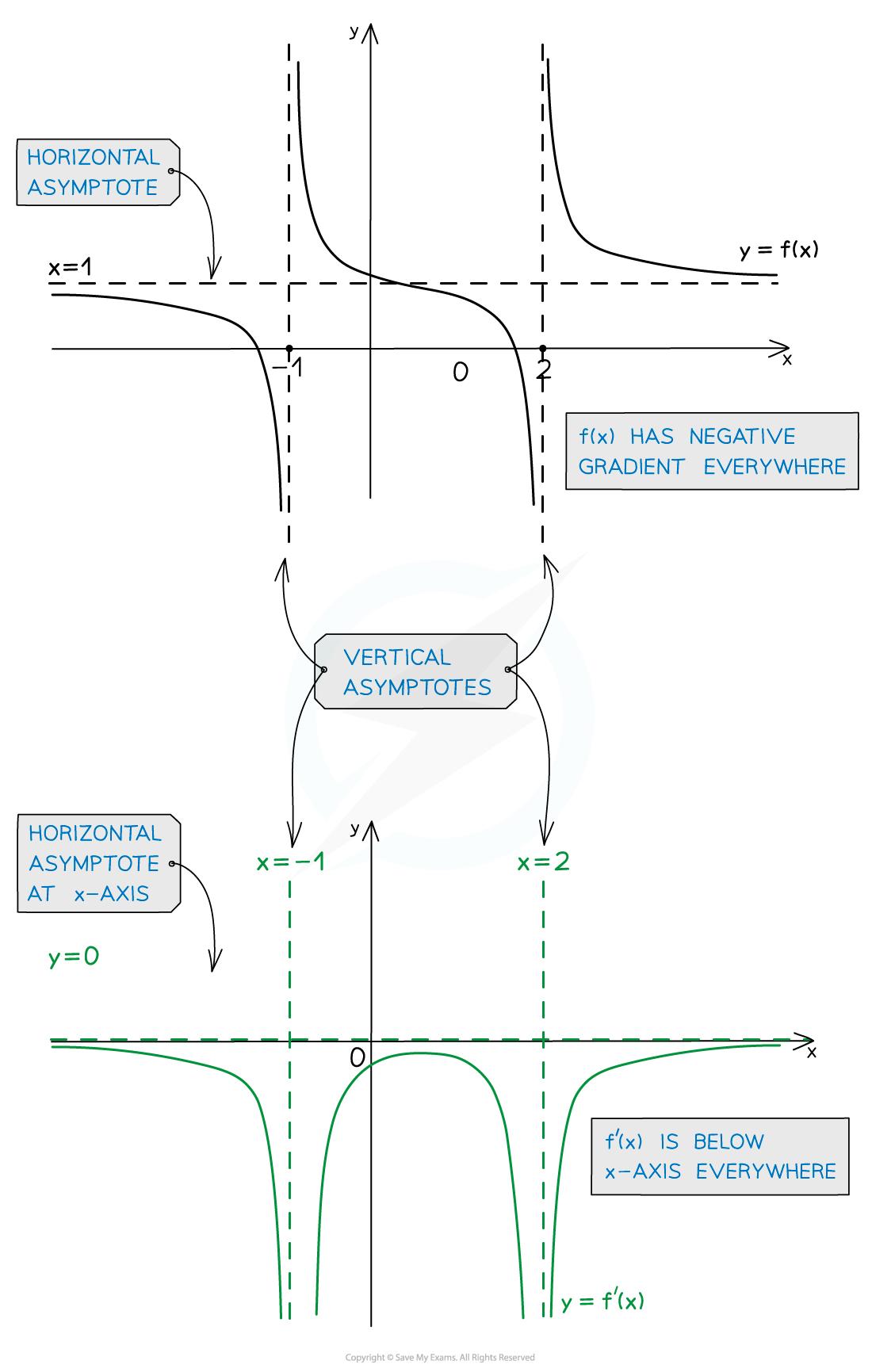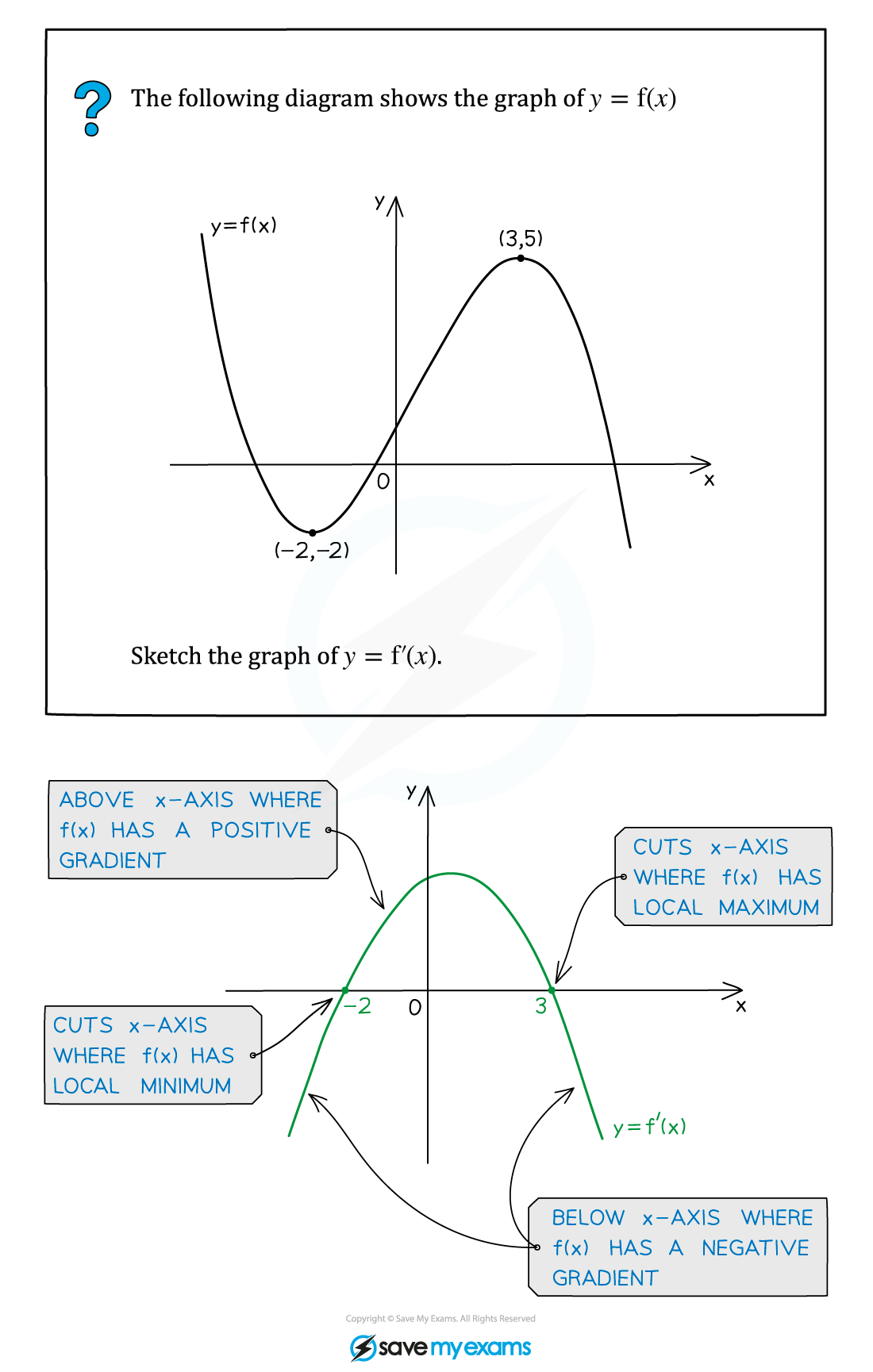# AQA A Level Maths: Pure复习笔记7.2.5 Sketching Gradient Functions

### Sketching Gradient Functions

#### How can I sketch a function's gradient function?

• Using your knowledge of gradients and derivatives you can use the graph of a function to sketch the corresponding gradient function
• The behaviour of a function tells you about the behaviour of its gradient function#### Exam Tip

• If f(x) is a smooth curve then f'(x) will also be a smooth curve.
• Take what you know about f'(x) (based on the table above) and then 'fill in the blanks' in between.
• If all you have is the graph of f(x) you will not be able to specify the coordinates of the y-intercept or any stationary points of f'(x).
• Be careful – points where f(x) cuts the x-axis don't tell you anything about the graph of f'(x)!

#### Worked Example